• c语言计算器代码程序设计报告《C语言课程设计》报告　设计题目：简单计算器设计　学院名称：电子与信息工程学院专业：计算机科学与技术班级：计科姓名：学号指导教师：　定稿日期：XX-5-28　一、设计题目　简单...
c语言计算器代码程序设计报告《C语言课程设计》报告　　设计题目：简单计算器设计　　学院名称：电子与信息工程学院专业：计算机科学与技术班级：计科姓名：学号指导教师：　　定稿日期：XX-5-28　　一、设计题目　　简单计算器设计　　二、设计要求　　在功能上功能尽量模拟windows操作系统中的计算器，系统界面不做强制要　　求。　　三、设计目的　　①通过本项课程设计，可以培养独立思考、综合运用所学有关相应知识的能　　力，能更好的巩固《C语言程序设计》课程学习的内容，掌握工程软件设计的基本方法，强化上机动手编程能力，闯过理论与实践相结合的难关！更加了解了c语言的好处和其可用性！同时增加了同学之间的团队合作精神！更加也体会到以后在工作中团队合作的重要性和必要性！　　②通过C语言课程设计，使学生了解高级程序设计语言的结构，掌握基本的程序设计过程和技巧，掌握基本的分析问题和利用计算机求解问题的能力，具备初步的高级语言程序设计能力。为后续各门计算机课程的学习和毕业设计打下坚实基础。　　四、设计思想及过程　　①由于需要使功能尽量模仿Windows下的计算器，而很多功能要以图形界面　　实现，故用BorlandC++Builder编写。所以用的是面向对象程序设计。但因为这是C语言的课程设计，所以除了面向对象的使用以外，所有语法都是按照C语言的格式编写。　　②采用工程模式，文件包括(运算主函数头文件，包括各运算符的操作以及其辅助函数)、(窗体类的声明头文件)、(工程初始化文件)、(代码主文件，包括各空间操作的函数)以及一些其它附属文件，包括dll动态链接库。　　③在参考了Windows里的计算器以后，决定在我的课程设计中实现：　　⒈0~9的数字输入功能　　⒉实现加、减、乘、整除、取模、阶乘、位运算左移、x的y次方、与、异或、x立方、x平方运算功能，二进制、八进制、十进制、十六进制　　转换功能，正负转换功能，退格、归零等功能。　　⒊数字显示最多为40位，超过即用科学表示法表示。⒋运算时因最高能对5000位的数字进行运算，故所有功能建立在自行编写代码的高精度运算基础上。　　④在按键操作中，先判断用户按的是数字键还是功能键。功能键分运算符键、进制转换键以及清空键。然后记录下来用户当前的按键，然后对上次按的功能键键进行运算。如按了1、+、5，再按任何功能键即对1+5进行运算。如果按的是进制转换键，就记录当前进制，则在以后每次操作中，都将显示结果转换为当前进制再显示。　　如：以下是按5以及*号时的函数代码　　⑤对于高精度，采用结构体structhigh{intnum[MAXN];};的形式，数组的一个元素存一位。个位为num，将符号存在num之中。　　⒈对于高精度加法，先判断两个数符号，如果为异号，则进行高精度减法，否则将各位逐个相加，然后逐位进位，返回答案即可。　　⒉对于高精度减法，先判断两个数符号，如果为异号，则进行高精度加法，否则用大数减小数，逐个相减，然后逐位退位，判断符号、返回答案即可。　　⒊对于高精度乘法，用第二个数的各位乘以第一个数的各位。然后错位相加，相当于小学生摆竖式。最后将各位相乘的结果相加，逐位进位，判断符号、返回答案即可。　　⒋对于高精度整除，采用各位从0~9枚举形式，如果此位与0~9相乘刚好小于等于相应数字，则为此位答案为这个数。最后返回答案即可。　　⒌对于高精度取模，则只需求出高精度整除，然后将数字减去整除的结果即可。　　⒍对于n阶乘，做n次乘法，每乘一次，乘数减一。　　⒎对于左移，虽然是建立在位运算基础上，但其效果只是乘以二，所以只需高精度乘以二即可。　　⒏对于x的平方、立方、y次方，只需将x高精度相乘2次、3次或者更多次即可。　　⒐对于与运算，先将两个数转换为二进制，然后对每位进行与运算，最后转换回十进制即可。　　⒑对于异或运算，原理同与运算。　　⑥对于进制转换，采用除n取余法。将其转换为相应进制。　　⑦对于清零键，按按键功能对actNum、ans、nowSym等变量清空或者减少一位即可。　　⑧我对于程序容错性做了很大程度上的优化。对于超出位数的运算会跳出“数字太大”提示。对于各按键混合使用会导致的程序混乱用了极大程度上的优化，也就是说对于按键的随意性有很好的兼容性。比如说可以直接按2再按*然后按=，就会算出2的平方。　　⑨对于显示函数，先判断当前进制数，然后再将需要显示的数字转换为当前进制数再显示。如果位数超过40，则取前几位，采用科学计数法显示。　　⑩未能实现部分：小数部分。由于时间关系，未能实现复制粘贴数字功能。　　五、流程图　　C语言课程设计报告　　题目：设计一个简单计算器　　目录　　1.设计目的　　2.内容
展开全文• o p using System; using System.Collections.Generic; using System.ComponentModel; using System.Data; using System.Drawing; using System.Linq; using System.Text; using System.Windows.Forms;...
• 该楼层疑似违规已被系统折叠隐藏此楼查看此楼while(sign!='a'){//if(input[index]){for(inti=0;i<4;i++){if(firsttime==1)i=3;//提取第i+1个数字dexto=1;charnum_str[INPUT_LEN]={'+'};while(input[index]){if...
该楼层疑似违规已被系统折叠 隐藏此楼查看此楼while(sign!='a'){//if(input[index]){for(int i=0;i<4;i++){if(firsttime ==1)i=3;//提取第i+1个数字dexto=1;char num_str[INPUT_LEN]={'+'};while(input[index]){if(isdigit(input[index]))num_str[dexto++]=input[index++];elseif((input[index]=='+'||input[index]=='-')&&dexto==1)num_str[dexto-1]=input[index++];else if(dexto>1)break;else index++;}if(input[index]=='.')if(isdigit(input[index+1])){num_str[dexto++]=input[index++];while(input[index])if(isdigit(input[index]))num_str[dexto++]=input[index++];else break;}num[i]=atof(num_str);if(input[index]=='\0'){for(;i<4;i++)sign[i]='a';break;}//提取第i+1个符号while(input[index]){switch(input[index]){case'+':case'-':case'*':case'/':case'%':case'^':sign[i]=input[index++];goto outofwhile;break;default:index++;continue;}}outofwhile:;}//提取数字符号结束firsttime =1;//}//判断结束结束//将数字进行计算if(sign=='+'||sign=='-'){//如果s0是加减则:if(sign=='+'||sign=='-'||sign=='a'){//如果s0,s1都一级num=singlecalculate(num,sign,num);num=num;num=num;sign=sign;sign=sign;sign=sign;}else{if(sign=='^'){//如果s0一级s1是^则num=singlecalculate(num,sign,num);num=num;sign=sign;sign=sign;}else if(sign=='^'){//如果s2是^且s1为二级num=singlecalculate(num,sign,num);sign=sign;}else{//如果s2为二级或1级则num=singlecalculate(num,sign,num);num=num;sign=sign;sign=sign;}}}else{//如果s0不是加法则if(sign=='^'&&sign!='^'){//如果s1是^则num=singlecalculate(num,sign,num);num=num;sign=sign;sign=sign;}else{//如果s0是二级或以上s1是二级或以下则：num=singlecalculate(num,sign,num);num=num;num=num;sign=sign;sign=sign;sign=sign;}}//num=singlecalculate(num,sign,num);/*printf("1:%lf\n",num);printf("2:%lf\n",num);printf("3:%lf\n",num);printf("4:%lf\n",num);printf("s1:%c\n",sign);printf("s2:%c\n",sign);printf("s3:%c\n",sign);printf("s4:%c\n",sign);*/}//大循环结束return num;}double singlecalculate(double num1,char sign,double num2){switch(sign){case'+':num1+=num2;break;case'-':num1-=num2;break;case'*':num1*=num2;break;case'/':num2==0?printf("式子包含有除数为0，请检查\a"):num1/=num2;break;case'%':num2==0?printf("式子包含有除数为0，请检查\a"):num1=(int)num1%(int)num2;break;case'^':num1=pow(num1,num2);break;}return num1;}程序我自己测试,没发现什么问�
展开全文• 计算器c源代码，非常好的源代码，可已参考 这是一个用c语言实现的完成计算器功能的程序，主要包括加、减、乘、除。
• 前段时间我自己写的一个，你看看，先是电路图，后面是代码图是用protues画的，有什么不懂加我QQ478769652//程序测试通过//能使用的功能：输入K1(+，-，*，/)K2，按=得到结果，然后按on/c或0重新开始//不能输入K1+K2+...
前段时间我自己写的一个，你看看，先是电路图，后面是代码图是用protues画的，有什么不懂加我QQ478769652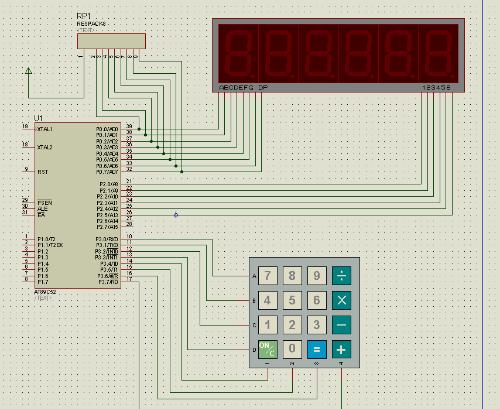//程序测试通过//能使用的功能：输入K1(+，-，*，/)K2，按=得到结果，然后按on/c或0重新开始//不能输入K1+K2+K3或者类似的输入，这种输入导致程序出错//计算除法的时候不能显示小数，如5/3得到的结果是1#includeunsigned char code tab={0x3f,0x06,0x5b,0x4f,0x66,0x6d,0x7d,0x07,0x7f,0x6f};sbit P2_0=P2^0;sbit P2_1=P2^1;sbit P2_2=P2^2;sbit P2_3=P2^3;sbit P2_4=P2^4;sbit P2_5=P2^5;sbit P3_4=P3^4;sbit P3_5=P3^5;sbit P3_6=P3^6;sbit P3_7=P3^7;long int n=0;unsigned int flag;void display();void delay();void keyboard();main(){//m1,m2分别保存第一次和第二次输入的两个数据//当m1，m2的输入值超过999999时，此时将其情0long int m1,m2;P2=0xff;while(1){//检测输入的第一个数据while(1){display();keyboard();if(n>999999) n=0;P3=0xf7;if(P3_7==0) {flag=1; while(!P3_7); m1=n; n=0; break; }P3=0xfb;if(P3_7==0) {flag=2; while(!P3_7); m1=n; n=0; break; }P3=0xfd;if(P3_7==0) {flag=3; while(!P3_7); m1=n; n=0; break; }P3=0xfe;if(P3_7==0) {flag=4; while(!P3_7); m1=n; n=0; break; }}//检测输入的第二个数据while(1){display();keyboard();if(n>999999) n=0;P3=0xf7;if(P3_6==0) {m2=n; break;}}//计算结果，将其保存在n中然后进行显示，注意当n的值太大超过4字节时，此时显示乱码while(1){if(flag==1) n=m1+m2;if(flag==2) n=m1-m2;if(flag==3) n=m1*m2;//由于类型原因，计算结果没有小数if(flag==4) n=m1/m2;if(n>999999) n=0;display();P3=0xf7;//计算完成之后，可以用on/c或者是0进行清0，然后进行下一轮计算if(P3_4==0||P3_5==0) {n=0;break;}}}}void keyboard(){//检测第一行的按键P3=0xfe;if(P3_4==0){n=n*10+7;while(!P3_4);}if(P3_5==0){n=n*10+8;while(!P3_5);}if(P3_6==0){n=n*10+9;while(!P3_6);}//检测第二行的按键P3=0xfd;if(P3_4==0){n=n*10+4;while(!P3_4);}if(P3_5==0){n=n*10+5;while(!P3_5);}if(P3_6==0){n=n*10+6;while(!P3_6);}//检测第三行的按键P3=0xfb;if(P3_4==0){n=n*10+1;while(!P3_4);}if(P3_5==0){n=n*10+2;while(!P3_5);}if(P3_6==0){n=n*10+3;while(!P3_6);}P3=0xf7;if(P3_5==0){n=n*10+0;while(!P3_5);}}//显示子程序void display(){P0=tab[n%10];P2_5=0;delay();P2_5=1;P0=tab[n/10%10];P2_4=0;delay();P2_4=1;P0=tab[n/100%10];P2_3=0;delay();P2_3=1;P0=tab[n/1000%10];P2_2=0;delay();P2_2=1;P0=tab[n/10000%10];P2_1=0;delay();P2_1=1;P0=tab[n/100000];P2_0=0;delay();P2_0=1;}void delay(){int i;for(i=0;i<500;i++);}
展开全文• 已结贴√问题点数：10回复次数：5 科学计算器(可实现带括号的浮点数四则运算)/*Name: 科学计算器(可实现带括号的浮点数四则运算)Copyright:Author:Date: 15-09-14 21:49Description:对近阶段所学的一个小总结，综合...
已结贴√问题点数：10 回复次数：5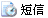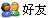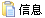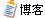科学计算器(可实现带括号的浮点数四则运算)/*Name: 科学计算器(可实现带括号的浮点数四则运算)Copyright:Author:Date: 15-09-14 21:49Description:对近阶段所学的一个小总结，综合了字符串与浮点数转换技术，利用逆波兰表达式，能顺利解决带括号的浮点数四则运算，并使用了文件操作，能够从文本文件中读入表达式并输出答案。算法思路：从文件读入计算表达式，将计算表达式转换为逆波兰表达式，然后计算出逆波兰表达式的值，最后输出答案。*/#include#include#include#include#define MAXSIZE 200void Translate(const char str[], char exp[]);//转换为逆波兰表达式double CompValue(const char *exp); //求逆波兰表达式的值double CharToDouble(const char *str); //将数字字符串转换为浮点数void GetStr(char *str); //读取计算式void ShowAnswer(const char *str, double answer); //输出答案int main(void){char str[MAXSIZE], exp[MAXSIZE];double answer;GetStr(str);puts(str);Translate(str, exp);puts(exp);answer =  CompValue(exp);ShowAnswer(str, answer); //输出答案return 0;}void GetStr(char *str) //读取计算式{FILE *fp;int i = 0;if ((fp=fopen("E:\\编程\\源代码基地\\c\\学习程序\\科学计算器计算式.txt", "rb")) == NULL){fprintf(stderr, "Error opening file.");exit(1);}rewind(fp);while ((str[i] = fgetc(fp)) != EOF){if (str[i] == ' ')//去掉多余的空格{i--;}i++;}str[i++] = '#';str[i] = '\0';fclose(fp);}void ShowAnswer(const char *str, double answer) //输出答案{FILE *fp;if ((fp=fopen("科学计算器计算式.txt", "a+b")) == NULL){fprintf(stderr, "Error opening file.");exit(1);}fprintf(fp, " = %f ", answer);fprintf(stdout, "%s = %f\n", str, answer);fclose(fp);}void Translate(const char str[], char exp[]) //转换为逆波兰表达式{char stack[MAXSIZE];int i = 0, k = 0, top = -1;while (str[i] != '#'){if (str[i] == '(') //直接将'('入栈{stack[++top] = str[i++];}else if (str[i] == ')'){while (top >= 0 && stack[top] != '(') //将'('之前的符号出栈，并存储到逆波兰表达式{exp[k++] = stack[top--];}if (top < 0) //'('不足，即有多余的')'{puts("'('不匹配\n");exp[k++] = '\0';return ;}top--;//去掉 '('i++;}else if (str[i] == '+' || str[i] == '-'){while (top >= 0 && stack[top] != '(') //如果有'('，将'('之前的符号出栈，否则所有符号出栈，并存储到逆波兰表达式{exp[k++] = stack[top--];}stack[++top] = str[i++]; //将新的运算符号入栈}else if (str[i] == '*' || str[i] == '/'){while (top >= 0 && (stack[top] == '*' || stack[top] == '/')) //将'*'和'/'之前的符号出栈，并存储到逆波兰表达式{exp[k++] = stack[top--];}stack[++top] = str[i++]; //将新的运算符号入栈}else{while ((str[i] >= '0' && str[i] <= '9') || str[i] == '.') //将浮点数直接存储到逆波兰表达式{exp[k++] = str[i++];}exp[k++] = '#'; //增加一个浮点数结束符号，以便正确提取浮点数}}while (top >= 0) //将栈中所有运算符号存储到逆波兰表达式{if (stack[top] == '(') //有多余的'('{puts("'('不匹配\n");exp[k++] = '\0';return ;}exp[k++] = stack[top--];}exp[k++] = '\0';}double CompValue(const char *exp) //求逆波兰表达式的值{char tempStr[MAXSIZE];double stack[MAXSIZE];int i = 0, k = 0, top = -1;while (exp[i] != '\0'){if (exp[i] >= '0' && exp[i] <= '9'){k = 0;while (exp[i] != '#'){tempStr[k++] = exp[i++];}tempStr[k] = '\0';stack[++top] = CharToDouble(tempStr);i++; //跳过'#'}else{switch (exp[i++]) //将计算结果入栈，并退出多余的数字{case '+' : stack[top-1] += stack[top];break;case '-' : stack[top-1] -= stack[top];break;case '*' : stack[top-1] *= stack[top];break;case '/' : if (stack[top] != 0){stack[top-1] /= stack[top];}else{puts("除零错误\n");return 0;}break;}top--; //退出多余的数字}}return stack[top];}double CharToDouble(const char *str) //将数字字符串转换为浮点数{double sumInt = 0, sumDec = 0, e = 1;int i = 0;while (str[i] >= '0' && str[i] <= '9'){sumInt = sumInt * 10 + str[i++] - '0';}if (str[i] == '.') //如果含小数，处理小数部分{while (str[++i] != '\0'){sumDec = sumDec * 10 + str[i] - '0';e *= 10;}}return sumInt + sumDec / e;}
展开全文• 说下思路和代码。#include int main() { double num1 = 0； //输入1 double num2 = 0； //输入2 . 0； } 写个简单易懂的，你7a686964616fe59b9ee7ad9431333332633631操作计算器.请各位大神帮忙编译一个简单计算器的...
• 自编小程序 供初学者参考 要过二级的可以下来看看 更多访问：http://www.liufuduan.com/
• 该楼层疑似违规已被系统折叠隐藏此楼查看此楼while(sign!='a'){//if(input[index]){for(inti=0;i<4;i++){if(firsttime==1)i=3;//提取第i+1个数字dexto=1;charnum_str[INPUT_LEN]={'+'};while(input[index]){if...
• 这是用C语言编写的计算器代码，可以借鉴学习一下，有错误的地方请改正
• C语言计算器（带小数，括号）超详细教程前言一、目标功能二、不带括号公式实现思路三、带括号公式实现思路总结 前言 最近开发嵌入式采集设备时需要实现对数据的公式处理，因为运算公式是可远程配置的，不能固定写死...
• 该楼层疑似违规已被系统折叠隐藏此楼查看此楼while(sign!='a'){//if(input[index]){for(inti=0;i<4;i++){if(firsttime==1)i=3;//提取第i+1个数字dexto=1;charnum_str[INPUT_LEN]={'+'};while(input[index]){if...
• VC++2008视频教程详解(含C++，类，MFC，API等)-郁金香2013网授共129讲，主要讲解了VC++等相关内容，比如C语言与C++区别、C++控制台程序、动态内存分配new和delet、初始化函数形参、异常抛出与捕获及处理、函数重载、...
• 本程序对于初学C语言的同学很有帮助，#include "iostream" using namespace std; int main( void ) { double numbera, numberb, result; char operate; try { //输入操作数和运算符 cout请输入第一个操作...
• C语言计算器_文库http://wenku..com/view/.html怎么学习编写计算机代码、程序谁在帮我c语言怎么入门c语言编程代码两种方法我写在一起，可以独立拆开。#includevoidfinda1(chara);voidfinda2(chara);...
• c语言写的一个计算器的源程序，可以看看，学学人家的编程风格
• 【欢迎下载】数据结构C语言编程作业 简单计算器 C语言程序 可运行
• ## C语言简易计算器

万次阅读 多人点赞 2018-08-21 14:22:22
C语言实现简易计算器： #include &lt;stdio.h&gt; void main() { int a,b; char c; //&amp;c存放符号 printf("请选择运算“+ - * /”\n"); while(1) { printf("输入运算符：&...
• ...............................................................................................................................
• 该文件中包含c语言写的一些小游戏，例如贪吃蛇、五子棋、俄罗斯方块、扫雷和计算器等，代码很好，希望对学习c言的同学编程提高有帮助
• C语言计算器 开发软件 Visual Studio 2017 代码如下： #define _CRT_SECURE_NO_WARNINGS #include <iostream> #include <stdio.h> #include <math.h> #include <malloc.h> void ...
• 2.5 C语言编程流程C语言编程流程a)解决什么问题b)怎么解决c)编写程序d)分析结果 2. 解决什么问题 ？比如说：我们如何打开、关闭一个计算器呢？3 那么我们怎么解决呢？我们可以通过命令行的方式打开、关闭计算器在...
• C语言中switch 的应用 程序思想 C++编程灵活简单我们只要有自己的对设计目标的整体思想其余的属于添砖 加瓦比如 此次计算器编程设计可能用到 switch 可能会达到简单操作领会直白等优 点可能会用到哪几种数据类型...
• ## C语言命令行编程

千次阅读 2020-02-14 18:01:31
通过ＳＹＳＴＥＭ，我们可以编程调用ＷＩｎｄｏｗｓ的一些列功能 CMD命令：开始－>运行－>键入cmd或command（在命令行里可以看到系统版本、文件系统版本） system( " gpedit.msc " ); 传递下列参数，就可以...
• 解题思路:注意事项:#include using namespace std;typedef long long LL;int n;string order;string Left,Right,oper;string Hex="10";//任意进制转换为十进制LL hexToTen(string num){LL HEX=atoll(Hex.c_str());...
• printf("********计算器********\n"); printf("****1.Add 2.Sub****\n"); printf("****3.Mul 4.Div****\n"); printf("**** 0.Exit ****\n"); printf("**********************\n"); } int Add(int x,int y) { ...
• 编程实现简单的计算器功能，要求用户按如下格式从键盘输入算式： 操作数1 运算符op 操作数2 计算并输出表达式的值，其中算术运算符包括：加（+）、减（-）、乘（）、除（/）、^(次幂）。 要求使其能进行浮点数的算术...
• 关于简易的整数计算机的设计制作算是c语言中较为基础的一道题，之前刚上课时老师也介绍过，但是我是初学，时间久了容易忘记，所以在此记录一下。 #include int main() { int a,op,b; scanf("%d%c%d",&a,&op,&b);...
• C语言优化方法 最简单的优化方法是借助prof工具判断程序的瓶颈在哪里,你必须判断出程序的那些部分消耗了大量资源. 一旦你判断出瓶颈(比如说执行上万次的循环),你所做的第一件事就是重新设计程序,减低循环次数....优化 语言 c 编译器...

# c语言计算器编程代码c语言 订阅